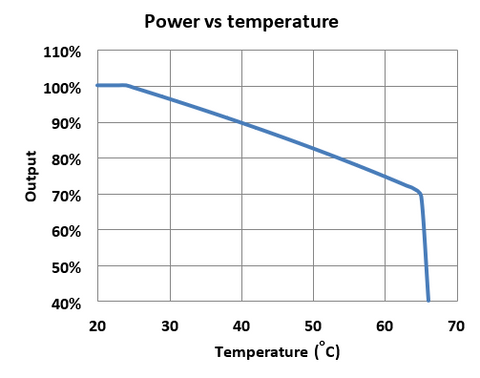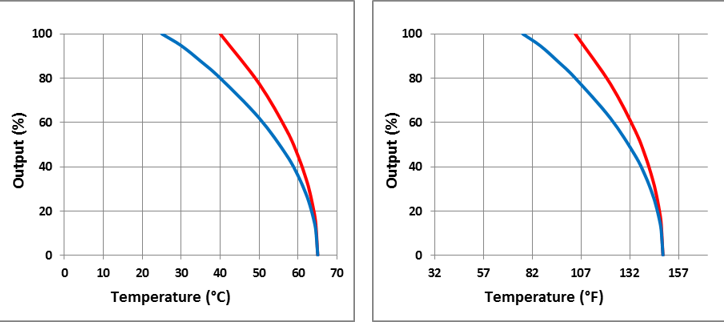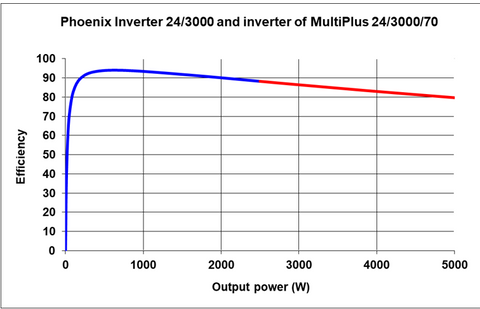# Output rating, temperature and efficiency

## OUTPUT RATING, OPERATING TEMPERATURE AND EFFICIENCY

1.    Inverters: continuous output rating as function of temperature

In our datasheets inverters, and the inverter function of Multis and Quattros, are rated at 25ºC (75ºF). On average, derating at higher temperatures is as shown below (see paragraph 4 for the theoretical background).

 Temperature Cont. output ºC ºF % Low temp. 100 25 77 100 30 86 96 35 95 93 40 104 90 50 122 82 60 140 75 65 149 69 High temp. 02. Battery chargers: continuous output rating as a function of temperature

In our datasheets battery chargers are rated at 40ºC (104ºF). The battery charger function of our Multis and Quattros is rated at 25ºC (77ºF). As explained in paragraph 4, derating for higher temperatures is approximately as follows:

 Blue curve: products rated at 25ºC (77ºF) Red curve: products rated at 40ºC (104ºF) Temperature Cont. output Temperature Cont. output ºC ºF % ºC ºF % 25 77 100 30 86 95 35 95 88 40 104 80 40 104 100 50 122 62 50 122 77 60 140 36 60 140 45 65 149 0 65 149 03. Power loss and efficiency as a function of load

As an example let us look at the Phoenix Inverter 24/3000 or MultiPlus 24/3000/70 (both products have the same inverter). These products use high frequency switching to generate a sinewave, which is then transformed to the required output voltage by two toroidal transformers. Toroidal transformers have a higher efficiency and less no load losses than the more common E-core transformers.

The measured efficiency curve is shown below:

 Output power (W) Dissipation (W) Efficiency (%) 0 20 0 10 20 33,3 20 20 50,0 30 20 59,9 50 20 71,3 100 21 83,0 200 22 90,1 400 28 93,5 800 52 93,9 1600 148 91,5 2500 333 88,3 3000 470 86,5 4000 820 83,0 5000 1270 79,7Recently a new product has been introduced: the MultiPlus-II 48/3000/35-32 (this is exactly the same product as the MultiGrid-II 48/3000/35-32)

The MultiPlus-II has only one toroidal transformer, and several other improvements to reduce no load power consumption. As can be seen from the table and graph below, this product is more efficient, especially at low load.

 Output power (W) Dissipation (W) Efficiency (%) 0 11 0 10 11 47,6 20 11 64,5 30 11 73,1 50 11 81,8 100 12 89,7 200 13 93,9 400 19 95,5 800 43 94,9 1600 139 92,0 2500 324 88,5 3000 461 86,7 4000 811 83,1 5000 1261 79,9

4. The theory behind the graphs of paragraph 1 – 3

4.1.  Operating temperature range

The operating temperature range may vary from one product to another.

The upper temperature is limited by the maximum operating temperature of certain components (for ex. semiconductors, electrolytic capacitors, relays).

The low temperature limit is mainly limited by the minimum operating temperature of semiconductors and electrolytic capacitors.

As has been shown in section 1 and 2, this does not mean that the full output rating is available over the whole temperature range.

Derating is needed at high ambient temperatures to prevent overheating of a.o. power semiconductors and transformers.

In general, output power cannot be increased at low temperatures due the maximum current rating of certain components (terminals, core saturation of filter chokes).

4.2.  Power loss and efficiency

Electric current generates heat in the conductor through which it flows. The basic formula to calculate the rate of heat generation, or power dissipation, is:

P = R x I²   (1)

where P stands for power (measured in Watts), R (Ohm) is the resistance of the conductor and I (Ampère, or Amps) the current.

What is interesting about this formula is that it shows that power dissipation increases with the square of the current. A resistance of for example 2 Ohm and a current of 10 Amps results in a dissipation of 2 x 10 x 10 = 200 W. Twice that current results in 4 times more heat generated: 2 x 20 x 20 = 800 W!

In power electronic circuits the situation is much more complicated: one has to do with DC losses and switching losses, losses in semiconductors and in high frequency transformers, etc. Very often, however, formula (1) appears to be a fairly good approximation of the overall losses in the circuit. The power dissipation P of the circuit can then be calculated by defining R as the overall resistance between input and output of the circuit and I as the output current.

An even better approximation is obtained if a factor is added to (1) to account for the no-load power consumption or no-load power dissipation. The no-load power consumption is the power dissipated by the circuit when it is switched on without any load connected. It is an important specification especially of inverters since in the long run it can drain a battery.

Taking into account the no-load power consumption results in the following formula:

Ploss = Po + R x I²out   (2)

Ploss is the total power dissipation in the product; Po is the no-load power dissipation (and by definition also the no-load power consumption); R is the “resistance” between input and output; and Iout the output current.

With formula (2) efficiency, an important specification of inverters, can be calculated:

η = 100 x Pout / (Pout + Ploss)   (3)

where η is the efficiency in % and Pout the output power (Pout = Vout x Iout).

At no-load conditions the output current Iout = 0 and the output power Pout = 0, so that:

Ploss = Po   (4)

and:

η = 0 / (0 + Po) = 0 %   (5)

In other words: the efficiency is 0 at no-load.

If there would be no power dissipation in the circuit (Ploss = 0), then:

η = 100 x Pout / (Pout + 0) = 100 %   (6)

In words: if an ideal circuit without any losses could be made, efficiency would be 100 %.

Formula (2) shows that with increasing load the losses at first do not increase very quickly because Po will still be very much larger than R x I²out as long as Iout is small. Efficiency will start at 0 and increase when the load increases.

But with the load increasing further, R x I²out will increase even faster and become larger than Po, so that Ploss will start increasing approximately with the square of the load or output current. After reaching a maximum, efficiency will therefore start decreasing as the load increases further.

4.3.  High temperature and derating

All power conversion products of Victron Energy are protected against damage due to overheating by temperature sensors placed on transformers and on the heatsink of the hottest semiconductors.

Inverters: When the power semiconductors and / or transformers reach a pre-set temperature, inverters will first show a temperature pre-warning, and if temperature increases further, the inverter will shut down. After cooling down, it will restart.

Battery chargers: When the power semiconductors and / or transformers reach a pre-set temperature, the output current will automatically be reduced to prevent a further increase in temperature.

The power semiconductors are the most critical, with a pre-set maximum heatsink temperature of approximately 80ºC (167ºF).

High frequency magnetics are in general limited to 100ºC, and toroidal transformers to 110ºC.

The derating curve resulting from maximum heatsink temperature will therefore show a steeper downward slope than the derating curve resulting from high frequency magnetics or a toroidal transformer.

In general the toroidal transformer is the limiting factor in our inverters and inverter/chargers, while semiconductors are the limiting factor in our battery chargers. This explains the difference between the curves shown in paragraph 1 and paragraph 2.

Knowing that, in case of forced cooling, the cooling capacity of a heatsink or transformer is proportional to the temperature difference between the heatsink/transformer and the cooling air flow, formula (2) can be restated as follows:

Iout = K x √(Tmax - ΔTo - Tamb)   (7)

where Iout is the output current; K is a constant; Tmax is the maximum heatsink or transformer temperature; ΔTo is the temperature rise of the heatsink or transformer due to the no-load power dissipation; and Tamb is the temperature of the cooling air flow.

Formula (7) shows that when ΔTo + Tamb = Tmax, Iout = 0.

In words: when the ambient temperature is so high that the no load power dissipation alone will cause the heatsink or transformer to reach the maximum temperature limit, the output current of the circuit is 0. Any output current would increase temperature beyond the maximum and result in shut down of the circuit due to overheating.

The derating formula (7) is applicable when the ambient temperature increases beyond the temperature at which the full output power is specified, in general 25ºC (77ºF) for inverters and 40ºC (104ºF) for battery chargers.

Why 25°C (77°F) for inverters? Inverters are very often used with intermittent loads. Short term power and peak power are therefore more important than the continuous rated power. Battery chargers on the contrary will regularly operate at maximum output current for several hours and are therefore rated for continuous operation at 40°C (104°F).